## 2013-06-26

### SBAC practice tests run-through – Part 1

At the end of May 2013, following its first pilot test for students, Smarter Balanced Assessment Consortium followed up on its previous releases of sample Common Core assessment questions with new batches for grades 3 through 8 and grade 11, in the form of online practice exams.

SBAC has since tweeted (read: crowed) unceasingly about these practice exams......and retweeted as well anyone else who’s mentioned the exams......in a positive light.

The below embedded quote is attributed to Sue Gendron, policy coordinator of SBAC: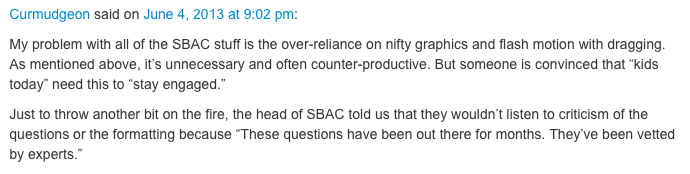We don’t know if this quote describes the previous questions or the new set.  Our email to Gendron, who holds a Ph.D., regarding the accuracy of this quote, went unanswered.  (The New York Times always seems to make repeated attempts to contact people; should we, too, or does that constitute harassment?)

Meanwhile, PARCC, whose official sample questions to date can be counted on one’s fingers, tweeted to us that it plans to release more samples this summer, something akin to a Friday news dump: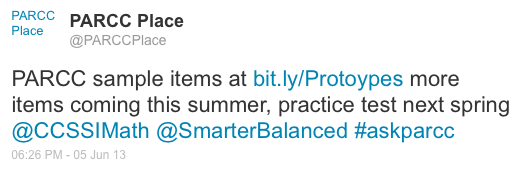Each SBAC practice exam has between 21 and 25 questions, and analysis of the questions has already begun.  Bowen Kerins, in the blog patternsinpractice, is taking a look at the Grade 11 questions.  In the blog mathmistakes, readers commented on a Grade 8 probability question, which we reprint below: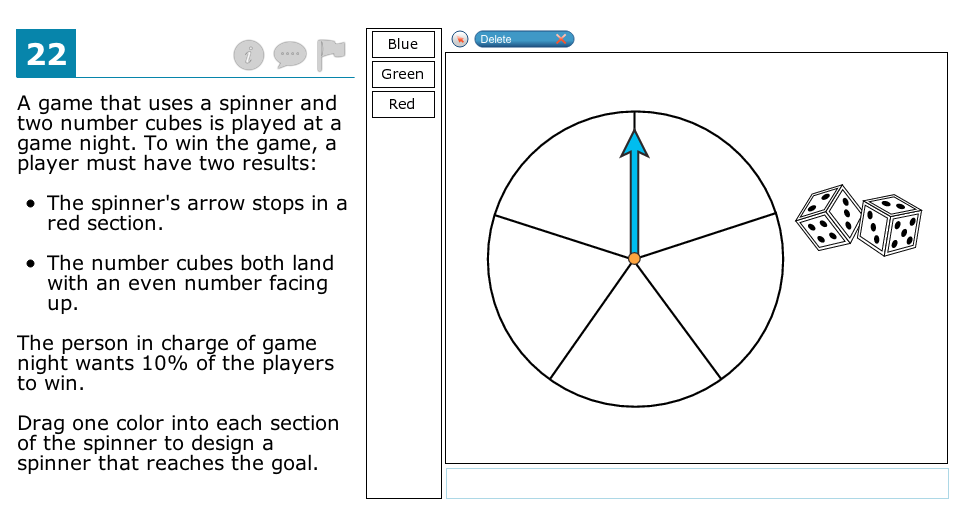We begin our own analysis by affirming that the mathematics behind this question is sound; simply put, the probability of independent events all occurring is the product of the probabilities of each event.

The SBAC question’s details and where it’s placed is another matter entirely.

It can be solved with a skill not covered in Common Core Grade 8 but in Grade 7, creating a tree diagram, which would need to be visualized in the student’s head or drawn on paper.  Tree diagrams for outcomes of “compound events” are found in CCSSI 7.SP.8a, which states, “8. Find probabilities of compound events using organized lists, tables, tree diagrams, and simulation.   a. Understand that, just as with simple events, the probability of a compound event is the fraction of outcomes in the sample space for which the compound event occurs.”  To be complete for this SBAC question, the tree diagram method would require students to draw 210 lines, determine the total number of outcomes (180=5 x 6 x 6), and then design the spinner in such a way that it creates 18 favorable outcomes, or 10% of the total.

However, the problem is far more easily solved by understanding how to calculate the overall probability of independent events, which is found in Common Core’s S-CP-2, a high school standard:

Understand that two events A and B are independent if the probability of A and B occurring together is the product of their probabilities, and use this characterization to determine if they are independent.

Tree diagrams, 210 separate lines in this particular problem, are somewhat unwieldy—an understatement—when problems become complex.  The second method is far easier.  So SBAC is attempting to test in Grade 8 a question that really should assess the understanding and application of a high school standard.

But that’s not all.  The question’s designers don’t fully align the question to the high school standard, either.  According to the question, the player wins the game if two outcomes both occur; presumably meeting the requirements on the spinner is one result and meeting the requirements on the dice is the other result.  However, these requirements don’t translate cleanly into the actual probability calculation.  That’s because this question doesn’t have just two, but three independent events.  The two dice are independent of each other.  (Is it possible the designers really meant to require “the sum of the pips on the dice is even”, which would change the game?)

So the mathematics needed to find the correct answer, three independent events, (two red sections = ⅖) x (one die = ½) x (other die = ½) = 1/10 or 10%, goes beyond the scope of Common Core.

All of this analysis so far goes to the mathematics behind the question, but there’s still more:

As Joshua Zucker commented on mathmistakes, “Those sure aren’t standard dice. I wonder how many of their sides are even?”  True that.  Standard dice have the opposite sides’ pips add to 7, and on one of these die you can see 3 & 4; the other 2 & 5.  But not only are they non-standard dice, they’re not even the same as each other: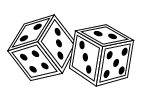Look closely.  If each die is oriented with the two pip side on top and the three pip side in front, the left die has the pips closest to each other on the right side; the right die has the pips closest to each other on the left.  Which means we really shouldn’t be making any assumptions about the rest of the dice’s sides.

The language in the question?  Confusing, ambiguous or omitting essential information, as is often the case.

More common language: number cubes are “dice”.  Or they should have numbers on the faces, not pips.

Necessary stated conditions: the dice are “fair”; the spinner is divided into five equal sections.

The question could be more succinctly asked: “You’re designing this game.  Drag a color into each section of the spinner so that the probability of a player winning is 10%.”

By Grade 3, students are supposed to be able to “solve two-step problems using the four operations. Represent these problems using equations with a letter standing for the unknown quantity.” See 3.OA.8

Does this SBAC question align to the standard?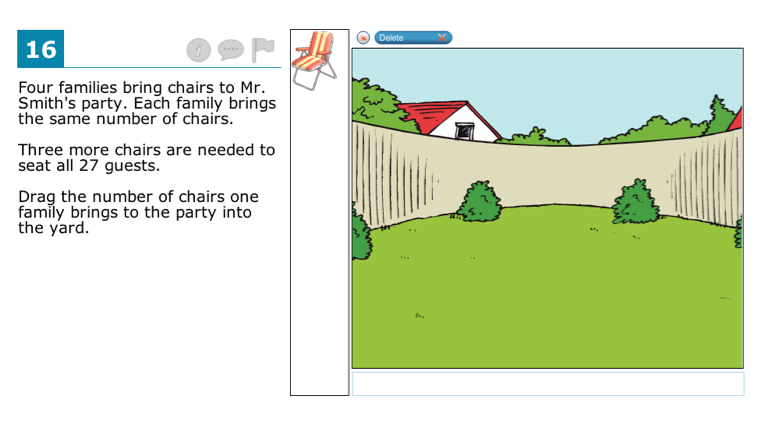One possible equation to represent this problem would be 4x + 3 = 27, where x is defined as the number of chairs.  It seems to us that (1) the second part of 3.OA.8 is putting algebra into Grade 3, and (2) there are more than two steps involved.

Either way, the strangeness of asking students to provide the answer by dragging folding chairs one-at-a-time onto an empty lawn overshadows both the questionable standard and the questionable alignment.  Next-generation assessments are supposed to be an advancement in sophistication and use of modern technology, not a sideshow.

The question also creates ambiguities, such as whether the 27 guests includes Mr. Smith, since it’s presumably his backyard.  Are the 27 guests all from the four families?  That would mean the average family size is over six.  Does Mr. Smith have no chairs at his house?  Where’s the food supposed to go?

In Common Core Grade 4, students study equivalent fractions. They also learn to multiply (whole number) x (fraction), but not (fraction) x (whole number).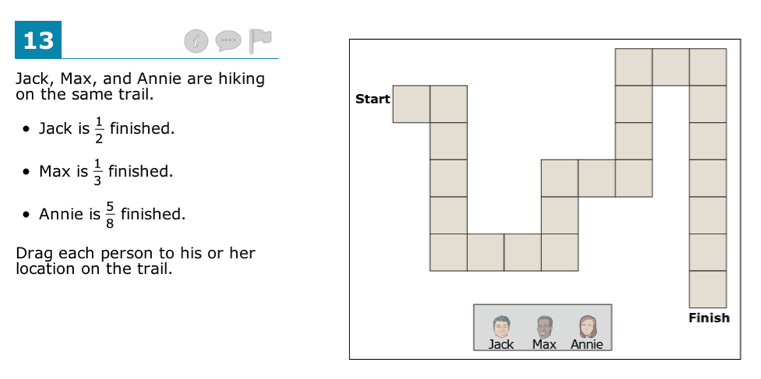How well will students apply one or the other skill, either of which can be used to solve this problem?

The question makes several design choices.  In questions of enumeration, or counting, one doesn’t start at square 1, rather, one must start at square 0, which in the problem is off the trail where the word Start is.  But finishing means landing on the last, or 24th square, not where the word Finish is.  It’s clear to us what the problem is asking, but in the details of this problem, the intent is muddied by poor design.

If “Jack is ½ finished”, then by counting, he would be on the 12th of 24 squares.  (If Jack had 24 cookies, and he was ½ finished, he would have eaten 12 cookies.)  However, if the problem is read literally, Jack should be standing exactly on the line with 12 squares behind him and 12 squares in front of him.

The answer can be found either by finding the equivalent fraction to ½ with a denominator 24, or 12/24, by multiplying 24 x ½, or by multiplying ½ x 24.  (The exam isn’t expecting the last approach, since that is found in the Grade 5 standards.  We don’t think the designer is expecting the second approach either.)

In another unfortunate design weakness, this problem requires students to count four times: the original count to determine there are 24 squares, for which there is no shortcut, and for the three answers.  There ought to be a better way to demonstrate fluency with equivalent fractions than by having students repeatedly demonstrate a kindergarten skill.

Finally, there’s that problem with language again.  Many weaker readers, even if they get the right answer for Jack, are going to stumble on the meaning of the expression “⅓ finished.”  “One-third of the way along the trail” is clearer.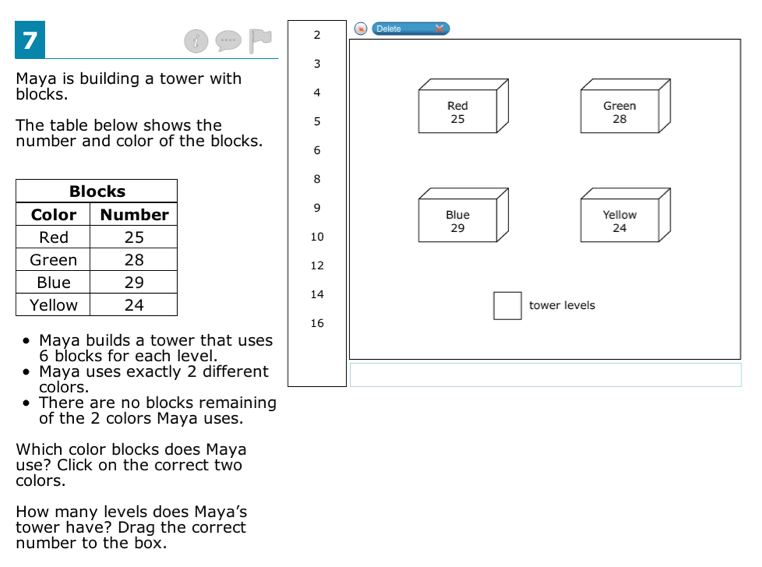For all of the words and pictures in this problem, and the three bulleted conditions, it boils down to a simple arithmetic question: Given four numbers, 24, 25, 28 and 29, determine the pair whose sum is evenly divisible by 6. Then find the quotient.

Once upon a time, that is exactly how the question would have been asked, but no longer.  Now it has to be “engaging”.  (Are fourth graders still interested in building blocks?)

The drawback in these interface questions, to repeat ourselves, is that with each element in a problem’s design, there is room for ambiguity and misleading language, much of it unnecessary.  For starters, the sentence “The table below shows the number and color of blocks” puts the word “number” before “color”, but the table reverses the words.  Far worse, the first sentence doesn’t state up front that Maya won’t be using all of the blocks, a key issue to understanding the problem.  Better wording might be, “Maya will use two different colors to build a tower of blocks.  She has four colors to choose from.”

We don’t expect Common Core assessments to abandon attempts at posing “real life” questions and return to questions framed in purely mathematical terms, but writers take heed: wording and the order of directives matter.

In an SBAC-specific interface design decision, many questions have two related parts separated by a vertical list of draggable numbers in a rectangle.  We don’t know why they chose this quirky design.  In this problem, the quotient (the number of levels) must be chosen from a list of 11 non-consecutive whole numbers.  Genuinely odd.

Common Core’s Standards for Mathematical Practice #3 begins: “Construct viable arguments and critique the reasoning of others.”  The SMPs deserve a blog of their own, but #3 stands out from the others in that it is not purely mathematical.  There’s talk among teachers of bringing the Socratic method into kindergarten classes, which gives new meaning to “paper chase”.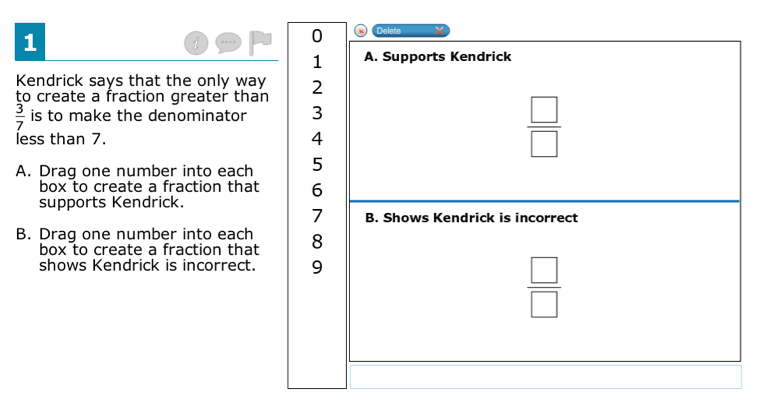“Support/don’t support the conjecture” format questions like this one appear repeatedly in the SBAC sample tests.  Andrew Stadel reviewed a Grade 6 question of this type as part of a nice assessment comparison done in a video format.  Straw poll: should ccssimath.blogspot.com use a video format?

Unfortunately, this SBAC question by its wording (and the others, as well) leads students down a mathematically indefensible path.  In mathematics, one does not prove a conjecture by one or numerous consistent examples, but one can disprove a conjecture by a single counterexample.

The weakness of this particular question is found by considering together both the conjecture’s absolute nature (“the only way”) and the wording in part A.  Students can write 3/6 (or many other choices) as the answer to part A, which is greater than 3/7, but it doesn’t “support” Kendrick’s conjecture in the mathematical sense of being one step on the way to completing a proof (described in SMP #3 thusly: “mathematically proficient students...make conjectures and build a logical progression of statements to explore the truth of their conjectures.”)  We shouldn’t be forcing fourth grade students to make or support fallacious arguments without understanding the big picture.

The question is simply asking students to “show how it possible to create a fraction greater than 3/7 by making the denominator less than 7.  Also show how it is possible to create a fraction greater than 3/7 where the denominator is NOT less than 7.”  Students are being asked to provide examples and counterexamples, but not prove anything.  It's clunky and additionally confusing because Part B is akin to a double negative—as in the above question, too—but correct mathematics.

Common Core is supposed to be increasing rigor, not fostering bad math.  Smarter Balanced is, too.

1.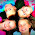Thank you so much for this thorough examination. I took the 3rd grade test and actually had to drag chairs over and was like, huh? Is this the robust rigor they were talking about?

2.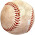I work at a Math learning/tutoring center. I have an opportunity to work with students from a number of surrounding school districts. These problems appear to me to be beyond the capabilities of the current grade level I have observed.

I understand and applaud the CCSS having higher expectations. My worry is that teachers will not have adequate training and time to improve students to these levels by the time CCSS is in full implementation.

Also, the 800-lb gorilla in the room is what happens with students whose test scores and grades are good/adequate who will now be adjudged as "Not Proficient" based on the CCSS' higher expectations.

3.This page answers a mystery of how CCSS can simultaneously be a grade or more down level from California 1997 and cause so much difficulty with test completion. Question 16 above explains it all. Supposedly a 3rd grade problem easily solved with what was 4th grade algebra in the old standard (now moved to 7th grade with CCSS). This is a cruel pointless joke. It's almost like testing a contractor to build a house without a hammer or screwdriver. I'm wondering what's going to happen when my tutor-trained 5th grader does solve these problems with algebra. Is he going to me marked wrong for not explaining the answer the same way as his algebra-naive classmates?

4.I completely agree about the trail problem. I would be genuinely confused about whether to put Jack on the 12th or 13th square (assuming that the interface doesn't allow any other options).

But I'm not so sure I agree about Kendrick's conjecture. I think this problem format is an interesting attempt to get at Grade 4 reasoning skills. The student who can both find a confirming instance of Kendrick's statement and also find a counterexample shows more reasoning ability than the student who can only do one of these. And finding a counterexample is proving something (namely that a statement is not true).

I don't think the word "support" has the specific mathematical meaning you attribute to it here; in fact most mathematicians would use the word the way it is used here, namely in the sense of confirming empirical evidence as distinct from mathematical proof. It's important for students to understand the difference between the two, and also to understand that a statement can have some confirming empirical evidence but still be false. A student who doesn't understand this point could have a harder time with this question, which means it could make it a good assessment. I'm not sure about this, but it seems worth trying to me.

5.m-m